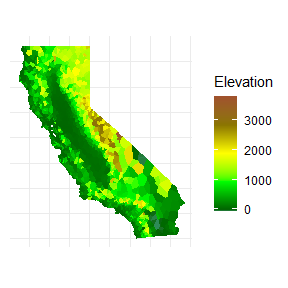# Voronoi Diagrams with ggvoronoi

#### 2022-08-25

With ggvoronoi we can easily draw Voronoi diagram heatmaps, with help from packages deldir and ggplot2. A Voronoi diagram draws the nearest neighbor regions around a set of points, and by specifying a fill argument we can turn that into a heatmap! Applications of Voronoi diagrams include:

• finding the one nearest neighbor to a given point
• interpolating a set of points without using a raster
• creating useful and appealing graphics

## Example 1: Voronoi Diagram Simulation

Let’s create some data to use as an example.

library(ggvoronoi)
set.seed(45056)
x <- sample(1:200,100)
y <- sample(1:200,100)
points <- data.frame(x, y,
distance = sqrt((x-100)^2 + (y-100)^2))
circle <- data.frame(x = 100*(1+cos(seq(0, 2*pi, length.out = 2500))),
y = 100*(1+sin(seq(0, 2*pi, length.out = 2500))),
group = rep(1,2500))

ggplot(points) +
geom_point(aes(x,y,color=distance)) +
geom_path(data=circle,aes(x,y,group=group))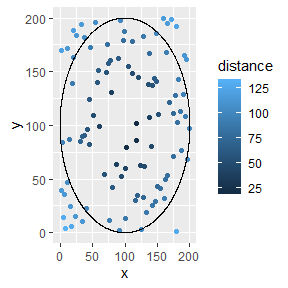All this data has is a set of x and y coordinates, along with the euclidean distance from the center of the circle which we will use to color the Voronoi diagram.

ggvoronoi can use this set of points to quickly plot a Voronoi diagram, working with the ggplot2 framework:

ggplot(points) +
geom_voronoi(aes(x,y,fill=distance))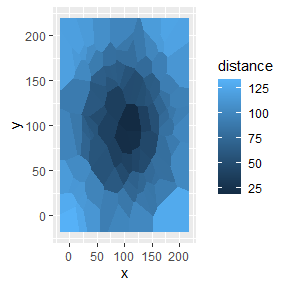Additionally, we can use the stat_voronoi function directly to specify which ggplot 2 geom we would like to use. This is most useful when we are interested in plotting only the borders of the diagram:

ggplot(points,aes(x,y)) +
stat_voronoi(geom="path") +
geom_point()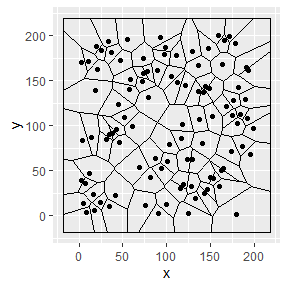This last plot brings up and interesting point: the deldir package creates the voronoi diagram with an automatic bounding box (otherwise it would be infinitely large!). But, we can specify our own bounding box to shrink or enlarge the area.

The outline argument must have these parameters:

• first column is x/longitude
• second column is y/latitude
• optional column “group”

Or you can feed it any SpatialPolygonsDataFrame!

For this example, we will use the circle included with ggvoronoi.

ggplot(data=points, aes(x=x, y=y, fill=distance)) +
geom_voronoi(outline = circle)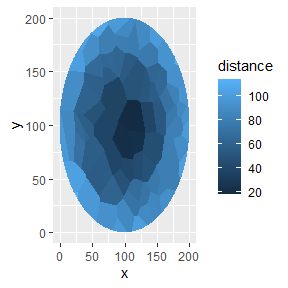Finally, you can add whatever you want with your knowledge of ggplot2!

ggplot(points,aes(x,y)) +
geom_voronoi(aes(fill=distance),outline=circle,
color="#4dffb8",size=.125) +
theme_void() +
coord_fixed()
#> Warning: It is deprecated to specify guide = FALSE to remove a guide. Please
#> use guide = "none" instead.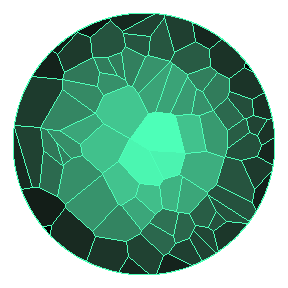## Example 2: Spatial Analysis with Oxford Bike Racks

For this example, we’ll be using the locations of each bike rack in Oxford, Ohio. Note that ggvoronoi is limited to the 2d euclidean distance calculations from the deldir package at the moment. As such, using longitude and latitude will result in approximate Voronoi regions, but with high sample size or a small area on the globe ggvoronoi still produces a useful (and near-exact) result!

Our goal here is to use a Voronoi diagram to locate the closest bike rack to points of interest in Oxford, OH. Being the hometown of Miami University, there is a lot of bike traffic in Oxford.

First we need to download a map of Oxford, using the ‘ggmap’ package. Note to download this yourself, you will need to supply your a Google Maps API key. For convenience, the ‘oxford_map’ object has been saved as a data file in the package, so feel free to skip this step and move to the next block of code!

library(ggmap)

oxford_map <- get_googlemap(center = c(-84.7398373,39.507306),zoom = 15,key="your_api_key")

Using this ggmap object we can make a map of Oxford in ggplot2.

bounds <- as.numeric(attr(oxford_map,"bb"))

map <- ggplot(data=oxford_bikes,aes(x,y)) +
geom_blank() +
inset_ggmap(oxford_map) +
xlim(-85,-84)+ylim(39,40)+
coord_map(ylim=bounds[c(1,3)],xlim=bounds[c(2,4)]) +
theme_minimal() +
theme(axis.text=element_blank(),
axis.title=element_blank())

Now that we have the base layer, we just need to add the diagram.

map + geom_path(stat="voronoi",alpha=.085,size=.25) +
geom_point(color="blue",size=.25)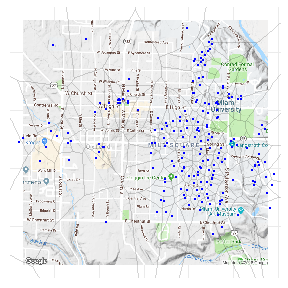Here we can see each bike rack along with the Voronoi region surrounding it. So, given a bike rack, the region surrounding it is the area in Oxford for which that is the closest bike rack. But what if we want to utilize this, not just look at it?

Using voronoi_polygon, we can build a Voronoi diagram as a SpatialPolygonsDataFrame. The voronoi_polygon function takes in:

• data: a data frame (will need at least 2 numeric columns)
• x: dataframe column name or index for the x variable
• y: dataframe column name or index for the y variable
• outline: a data.frame or SpatialPolygonsDataFrame with a map outline
ox_diagram <- voronoi_polygon(oxford_bikes,x="x",y="y")

This function is valuable in 2 cases: when there is spatial analysis to perform and when you are making a diagram of a large set of points.

Now, lets take a point of interest in Oxford, say Mac & Joes, a popular restaurant/bar. Google Maps can give us directions there, but there is no place to chain up a bike. So, lets use the diagram!

Create a point with Mac & Joes’ location:

library(sp)
mac_joes <- SpatialPointsDataFrame(cbind(long=-84.7418,lat=39.5101),
data=data.frame(name="Mac & Joes"))

Then, overlay the point on our Voronoi diagram,

mac_joes %over% ox_diagram
#>           x       y name
#> 1 -84.74177 39.5106 <NA>

There we have the coordinates of the closest bike rack to Mac & Joes!

Let’s plot the map again.

First, plot the Voronoi regions using the SpatialPolygonsDataFrame. Next, zoom into the area of interest (Uptown Oxford). Then, plot Mac & Joes with a red point. Plot the rest of the racks for visual comparison. Lastly, find the closest bike rack and drop a blue point.

map + geom_path(data=fortify_voronoi(ox_diagram),aes(x,y,group=group),alpha=.1,size=1) +
coord_map(xlim=c(-84.746,-84.739),ylim=c(39.508,39.514)) +
geom_point(data=data.frame(mac_joes),aes(long,lat),color="red",size=2) +
geom_point(size=1.5,stroke=1, shape=21,color="black",fill="white") +
geom_point(data=mac_joes %over% ox_diagram,aes(x,y),color="blue",size=2)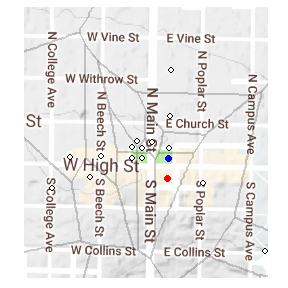So, we can see if you’re headed to Mac & Joes for lunch you’re better off using the bike rack across High Street than the one on South Poplar.

## Example 3: Altitude of California

Here, we will be demonstrating the heatmap capabilities of ggvoronoi. California has one of the most varied geographies of any US state. So, we’ll use ggvoronoi to create a heatmap of the altitude of California!

First, subset out the data and outline.

library(dplyr)

california <- map_data("state") %>% filter(region == "california")
ncdc.cali <- ncdc_locations %>% filter(state=="CA")

Next, lets plot the data we just gathered. We’ll create a base layer, containing just the theme and design information we’ll need. Then, we’ll add some points and the California border to the plot.

cali_map <-
ggplot(data=ncdc.cali,aes(x=long,y=lat)) +
colors=c("seagreen","darkgreen","green1","yellow","gold4", "sienna"),
values=scales::rescale(c(-60,0,1000,2000,3000,4000))) +
colors=c("seagreen","darkgreen","green1","yellow","gold4", "sienna"),
values=scales::rescale(c(-60,0,1000,2000,3000,4000))) +
coord_quickmap() +
theme_minimal() +
theme(axis.text=element_blank(),
axis.title=element_blank())

cali_map +
geom_point(aes(color=elev),size=.01) +
geom_path(data=california,aes(long,lat,group=group),color="black")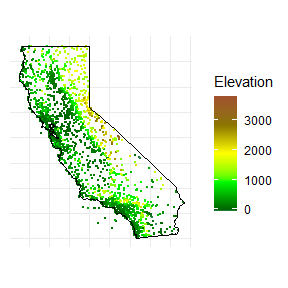We can see each point is colored based on the elevation. Luckily, ggvoronoi will let us smooth out these points without using a raster. Now, we’re ready to create the heatmap!

cali_map +
geom_voronoi(aes(fill=elev),outline=california)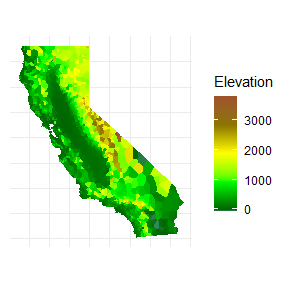Here we can see everything from Death Valley (dark green) to Mount Whitney, the highest point in the state (dark brown)!

In the second example, we used the voronoi_polygon function for spatial analysis. But, we’ll repeat this third example using voronoi_polygon to demonstrate how to build a heatmap using geom_polygon. This is useful when we have many points, as you only want to calculate the Voronoi regions once instead of each time you create a plot.

california <- map_data("state") %>% filter(region == "california")

ncdc.cali <- ncdc_locations %>% filter(state=="CA")

cali.voronoi <- voronoi_polygon(data=ncdc.cali,
x="long",y="lat",
outline=california)

Perform spatial analysis here, if necessary.

Once you are done with analysis and ready to plot, use fortify_voronoi.

cali.voronoi <- fortify_voronoi(cali.voronoi)

ggplot(cali.voronoi) +
geom_polygon(aes(x=long.x, y=lat.x ,fill=elev,
group=group, color=elev), size=0) +
axis.title=element_blank())